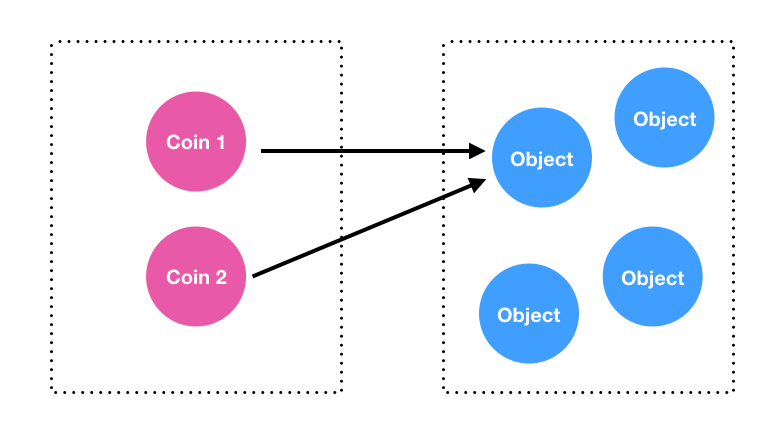JavaScript 基本型別 (Primitives) 內的資料，會是以純值的形式存在 ( `String``Number``Boolean``Null` 以及 `Undefined` )，而物件型別 (Object) 指的是可能由零或多種不同型別 (包括純值與物件) 所組合成的物件。

## 基本型別

``````var a = 10;
var b = 10;

// 在 JavaScript 判斷是否「相等」用 ===
console.log( a === b );      // true
``````

``````var a = 'Kuro';
var b = 'Kuro';
var c = 'Jack';

console.log( a === b );      // true
console.log( a === c );      // false
``````

## 物件型別

``````var obj1 = { value: 10 };
var obj2 = { value: 10 };
``````

.
.
.
.
.
.

``````var a = 10;
var b = 10;

console.log( a === b );      // true
``````

``````// 兩個 coin 的價值都是 10，但 coin1 與 coin2 卻不是同一個實體。
var coin1 = { value: 10 };
var coin2 = { value: 10 };

console.log( coin1 === coin2 );      // false

// 我在 coin1 畫了一個 X
coin1.cross = true;

// coin2.cross 當然不可能會有東西
console.log( coin2.cross );          // undefined
``````

## 變數的更新與傳遞

### 基本型別的更新與傳遞

``````var a = 10;
var b = a;

console.log( a );   // 10
console.log( b );   // 10
``````

``````a = 100;

// 變數 b 依然是 10，而變數 a 變成了 100
console.log( a );   // 100
console.log( b );   // 10
``````

### 物件型別的更新與傳遞

``````var coin1 = { value: 10 };
var coin2 = coin1;

console.log( coin1.value );       // 10
console.log( coin2.value );       // 10
``````

``````coin1.value = 100;

console.log( coin1.value );       // 100
console.log( coin2.value );       // 100
``````

`coin1.value` 的內容被更新了之後，連帶著 `coin2.value` 卻也跟著更新了。

``````console.log( coin1 === coin2 );    // true
````````````var coin1 = { value: 10 };
````````````var coin2 = coin1;
``````

``````coin1.value = 100;

console.log( coin1.value );       // 100
console.log( coin2.value );       // 100
``````

## JavaScript 是「傳值」或「傳址」？

``````var coin1 = { value: 10 };

function changeValue(obj) {
obj = { value: 123 };
}

changeValue(coin1);
console.log(coin1);   // ？
``````

「傳值」或「傳址」對大多數的開發者來說應該都不陌生，那麼「pass by sharing」又是什麼呢？

## Pass by sharing

「Pass by sharing」的特點在於，當 `function` 的參數，如 `function changeValue(obj){ ... }` 中的 `obj` 被重新賦值的時候，外部變數的內容是不會被影響的。

``````var coin1 = { value: 10 };

function changeValue(obj) {
obj = { value: 123 };
}

changeValue(coin1);
console.log(coin1);   // 此時 coin1 仍是 { value: 10 }
``````

``````var coin1 = { value: 10 };

function changeValue(obj) {
// 僅更新 obj.value，並未重新賦值
obj.value = 123;
}

changeValue(coin1);
console.log(coin1);   // 此時 coin1 則會變成 { value: 123 }
``````

## 結論

Regardless of usage concept of reference in this case, this strategy should not be confused with the “call by reference” discussed above. The value of the argument is not a direct alias, but the copy of the address.

``````var a = 10;
var b = a;

a = 100;

console.log(a);     // 100
console.log(b);     // 10
``````